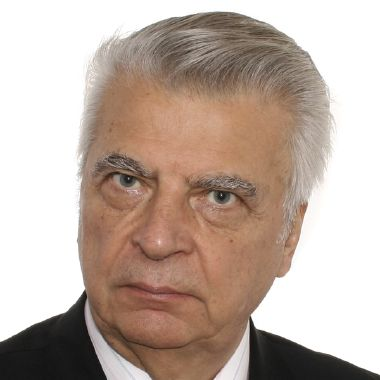Categories Pricing CorporateFree Textbook

# Stability Analysis via Matrix Functions Method

### Part II

0 Reviews
130
Language:  English
The monograph presents a generalization of the well-known Lyapunov function method and related concepts to the matrix function case within systematic stability analysis of dynamical systems.
Description
Preface
Content

The monograph presents a generalization of the well-known Lyapunov function method and related concepts to the matrix function case within the framework of systematic stability analysis of dynamical systems (differential equations). Applications are provided with stability issues of ordinary differential equations, singularly perturbed systems, and stochastic differential equations up to some applications to so-called real world situations.

The book is organized in five chapters. Each chapter is accompanied by numerous examples and notes on the locally related bibliography. This book is very innovative and systematically developed and rich on new ideas in contemporary stability theory.

Thus it can be recommended to any specialist in nonlinear dynamical systems and differential equations, both in deterministic and stochastic analysis.

One can hardly name a branch of natural science or technology in which the problems of stability do not claim the attention of scholars, engineers, and experts who investigate natural phenomena or operate designed machines or systems. If, for a process or a phenomenon, for example, atom oscillations or a supernova explosion, a mathematical model is constructed in the form of a system of differential equations, the investigation of the latter is possible either by a direct (numerical as a rule) integration of the equations or by its analysis by qualitative methods.

The direct Liapunov method based on scalar auxiliary function proves to be a powerful technique of qualitative analysis of the real world phenomena. This volume examines new generalizations of the matrix-valued auxiliary function. Moreover the matrix-valued function is a structure the elements of which compose both scalar and vector Liapunov functions applied in the stability analysis of nonlinear systems.

Due to the concept of matrix-valued function developed in the book, the direct Liapunov method becomes yet more versatile in performing the analysis of nonlinear systems dynamics.

The possibilities of the generalized direct Liapunov method are opened up to stability analysis of solutions to ordinary differential equations, singularly perturbed systems, and systems with random parameters.

The reader with an understanding of fundamentals of differential equations theory, elements of motion stability theory, mathematical analysis, and linear algebra should not be confused by the many formulas in the book. Each of these subjects is a part of the mathematics curriculum of any university.

In view of the fact that beginners in motion stability theory usually face some difficulties in its practical application, the sets of problems taken from various branches of natural sciences and technology are solved at the end of each chapter. The problems of independent value are integrated in Chapter 5.

1. Stability Analysis of Stochastic Systems
1. Introduction
2. Stochastic Systems of Differential Equations in General
3. Stability to Systems in Kats-Krasovskii Form
4. Stability to Systems in Ito’s Form
5. Applications
6. Notes
2. Some Models of Real World Phenomena
1. Introduction
2. Population Models
3. Model of Orbital Astronomic Observatory
4. The Power System Model
5. The Motion in Space of Winged Aircraft
6. NotesA. A. Martynyuk

Martynyuk is organizer and head of the Department of Processes Stability at the S.P. Timoshenko Institute of Mechanics of the National Academy of Sciences of Ukraine (NAS of Ukraine) since 1978.

He developed the method of Lyapunov matrix functions as applied to uncertain systems, systems with fuzzy information, and dynamic equations on time scales, non-classical theories of stability of motion, chaotic dynamics, and mathematical ecology.

As the editor of a series of scientific monographs "Stability and Control: Theory, Methods and Applications" (1995-2002) at the publishing house Gordon and Breach (UK) and Taylor and Francis (USA) he organized the work of scientists from many countries of the world to create the series consist with 22 volumes, which opened new opportunities for young scientists to continue research in many areas of research in the qualitative theory of equations.

He is currently focusing on the publication of a series of scientific monographs "Stability, Oscillation and Optimization of Systems" at the publishing house Cambridge Scientific Publishers (UK).

Martynyuk is the founder and editor-in-chief of the International Scientific Journal "Nonlinear Dynamics and Systems Theory", which is well known throughout the world.

He is the author (co-author) of a number of monographs in Russian, English and Chinese.

He is a doctor of physical and mathematical sciences, professor, academician of the NAS of Ukraine, laureate of the State Prize of Ukraine in the field of science and technology.

Martynyuk is based in Ukraine, but he actively communicates with experts from all over the world that are interested in stability theory and motion control in the study of real-world phenomena.